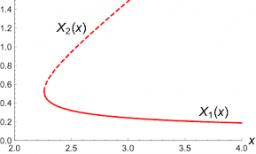# Domains: 4845

Specify function domains:
a / y = 2x-1
b / y = 5x / (2x + 1)
c / y = x2 / (x2-9)

a = R
b = R-{-1/2}
c = R-{-3,3}

### Step-by-step explanation:Did you find an error or inaccuracy? Feel free to write us. Thank you!

Tips for related online calculators
Are you looking for help with calculating roots of a quadratic equation?